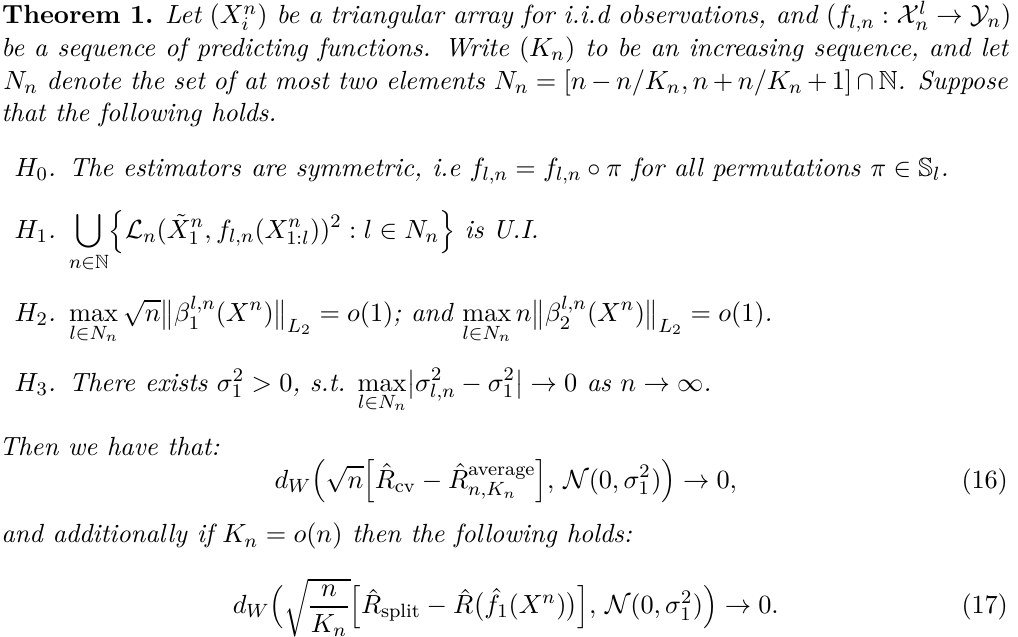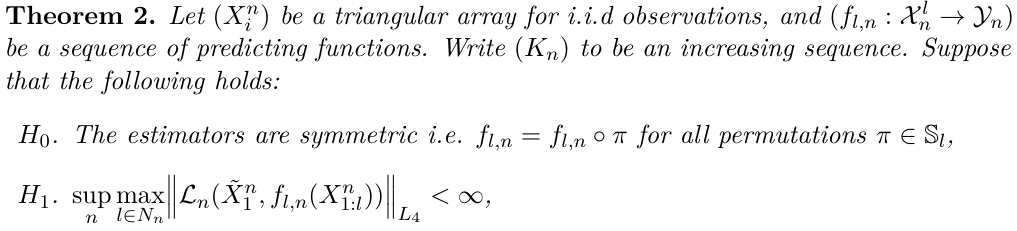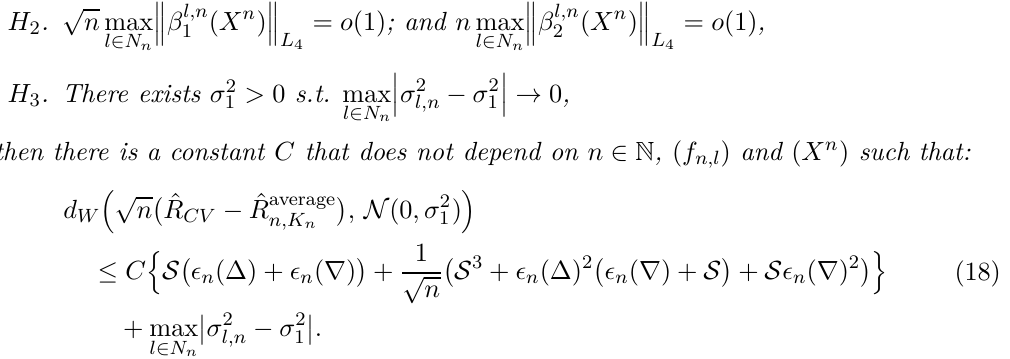# Asymptotics of Cross Validation

##### Posted on Dec 03, 2021

The theoretical properties of cross validation are still not well understood.

The paper study the asymptotic properties of the cross validated-risk for a large class of models.

• study its speed of convergence to the expected risk and compare it to the data splitting estimator $\hat R^{split}$

Under stability conditions, the paper establishes a CLT and Berry-Esseen bounds, which enables to compute asymptotically accurate CI.

Using their results, they paint a big picture for the statistical speed-up of cross validation compared to a train-set split procedure.

A corollary is that

• parametric M-estimators (or empirical risk minimizers) benefit from the “full” speed-up when performing cross-validation under the training loss.
• in other common cases, such as when the training is performed using a surrogate loss or a regularizer, the paper shows that the behavior of the cross-validated risk is complex with a variance reduction which may be smaller or larger than the “full” speed-up, depending on the model and the underlying distribution.

Allow the number of folds $K_n$ to grow with the number of observations at any rate.

There are at least two different quantities which may be seen as targets for the cross-validated risk estimator

• the average risk of the hypotheses learned on each fold
• the risk of the estimation procedure over replications of the dataset

confused, what does this mean?

## Main Results

• $(\cX_n), (\cY_n)$: two sequences of Borel spaces. Observations take values in $\cX_n$ and estimators in $\cY_n$
• $X^n := (X_i^n)_{i\in \bbN}$: a sequence of processes of i.i.d. random variables taking values in a Borel space $\cX_n$

think of estimators as (measurable) functions that map observations to an element of $\cY_n$

For all $n\in \bbN$, consider a sequence of estimators

$(f_{l, n}:\prod_{i=1}^l\cX_n\rightarrow \cY_n)_{l\in\bbN}$

The associated loss on an observation $x\in\cX_n$ is measured by a sequence of loss functions

$(\cL_n:\cX_n\times \cY_n\rightarrow\bbR)$

The goal is to approximate

$\bbE\left[\cL_n(X_0^n, f_{l_n,n}(X_1^n,\ldots, X_n^n))\right]$
• $(K_n)$: a non-decreasing sequence of integers
• $(B_i^n)_{i\le K_n}$: a partition of $[n] = {1,\ldots,n}$
• $\hat f_j(X^n)$: the estimator trained on $X_{[n]\backslash B_j^n}^n$ as

for a given hypothesis $f\in\cY_n$, define the risk

$R_n(f) := \bbE(\cL_n(\tilde X_1^n, f))$
• $b_n(i)$: the index of the partition element $i$ belongs to

compare the performance of the two following estimators of the predictive risk of an estimator

$\hat R_{cv} := \frac 1n\sum_{i=1}^n \cL_n(X_i^n, \hat f_{b_n(i)})$ $\hat R_{split} = \frac{K_n}{n}\sum_{i\in B_1^n}\cL_n(X_i^n, \hat f_1)$

Consider the risk of the ensemble estimator,

$\hat R_{n,K_n}^{average} := \frac{1}{K_n}\sum_{j\le K_n}R_n(\hat f_j)$

Its expected value is

$\bar R_{n, K_n} := \frac{1}{K_n}\sum_{j\le K_n}R_{n-\vert B_i^n\vert, n}$

confused, what is the difference? $\tilde X_0$ vs $\tilde X_1$?

When studying the asymptotics of the cross-validated risk estimator $\hat R_{cv}$, one can study its convergence to either $\hat R_{n,K_n}^{average}$ or alternatively to $\bar R_{n, K_n}$.For Berry-Esseen bound,## Example: Ridge Regression

Let $(Z_i)$ and $(Y_i)$ be a sequence of i.i.d. observations in respectively $\IR^d$ and $\IR$; and $\lambda\in\IR^+$ be a constant. Define $(\hat\theta_{l,n})$ be the following sequence of estimators:

$\hat\theta_{m,n}(X_{1:m}):=\arg\min_{\theta\in\IR^d}\frac 1m\sum_{i\le m}(Y_i-X_i^T\theta)^2 + \lambda\Vert \theta\Vert_{L_2}^2$

## Parametric Estimation

Thm 3 guarantees, under stability conditions, that the cross-validated risk converges $\sqrt{K_n}\sqrt{\frac{1}{1+2\frac{\rho}{\sigma_1^2+\sigma_2^2}}}$ faster than the simple split risk.

Use the term “full speed-up” to refer to the case where the cross-validated rosk converges $K_n$-times faster compared to the corresponding train-test split estimator, as if the folds were independent. This corresponds to $\rho=0$.

• $\rho > 0$: the cross-validated risk converges slower compared to the “full speed-up” case
• $\rho < 0$: the convergence is even faster

Published in categories Note